Reach Us+44-7482-875032
A New Number Theory - Algebra Analysis | OMICS International
Journal of Applied & Computational Mathematics
All submissions of the EM system will be redirected to Online Manuscript Submission System. Authors are requested to submit articles directly to Online Manuscript Submission System of respective journal.

# A New Number Theory - Algebra Analysis

Sonaglioni L*

Free Professionist, Italy

*Corresponding Author:
Sonaglioni L
Free Professionist, Italy
Tel: 388-0579470
E-mail: [email protected]

Received date: November 23, 2015; Accepted date: December 01, 2015; Published date:December 05, 2015

Citation: Sonaglioni L (2015) A New Number Theory - Algebra Analysis. J Appl Computat Math 4:267. doi:10.4172/2168-9679.1000267

Copyright: © 2015 Sonaglioni L. This is an open-access article distributed under the terms of the Creative Commons Attribution License, which permits unrestricted use, distribution, and reproduction in any medium, provided the original author and source are credited.

Visit for more related articles at Journal of Applied & Computational Mathematics

#### Abstract

The article proposes some analytical considerations about the 3d algebra, and the possibilities of an extension in 3d of some standard 2d analytical functions. It takes also in consideration some problems about the derivative and the integrals.

#### Keywords

Quaternions; Number theory; Operator theory; Algebra; Tensor methods

#### Three-Dimension

Let us consider the 3d space that can be represented by the tern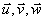as shown in Figure 1.

Figure 1: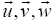are unit vector in the three-dimensional space.

A recently publication  have shown that the point P (a 3d number [x,y,z]) can be written as:

P =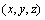=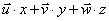and also, using the polar notation, as P =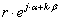where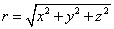the definition of the sum is:

1)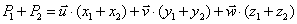[cartesian notation]

the definition of the product is:

2)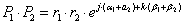[polar notation]

The two rules of above, and the transformations between the polar notation and the cartesian notation of the point P (and vice versa) give a 3d algebra definition as an extension of the sum and product of the 2d standard complex algebra.

What else can we say about this algebra?

First we have to observe that this algebra is commutative but not distributive, in fact:

3)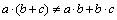, where a, b and c are 3 dimensions numbers as above.

This is because the transformations used for the definition of the product are not linear.

We can try to define the standard functions such as es, ln(s), cos(s), sin(s)

The problem can be seen as an extension of the same 2d complex functions.

If we extend es by the series definitions:

4)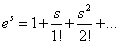Simulations show that ln(s) is not a biunique function unless 2.π for the arguments. I’ve found two distinct s values whose es defined as 4) is the same.

Example: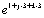= (-10.87282, 20.69650, 3.333867)

but also: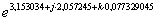= (-10.87282, 20.69650, 3.333867)

So we must define es as the standard extension of the 2d complex exponential function:

5)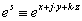= polar notation of: (exp(x), y, z)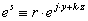where r=ex

In this case ln(s) is defined as the cartesian notation of: (ln(r), mod(a,2π), mod(b,2π)) and it is a unique function unless 2π for the angles arguments.

What about derivative of es (?) again, the algebra is not distributive, the limit: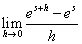h is a 3d number does not exist, for the same reason of the derivative of s2 (see below and code sample).

We can also define cos(s) and sin(s) as an extension of the standard 2d complex definitions, even that may be not clear the significance of these functions.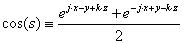And sin(s)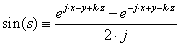The calculus of these definitions is simple, but the derivative does not exists, because derivative of es does not exist.

Also, simulations have shown that (for 3d numbers):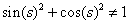So the definitions of sin(s) and cos(s) for 3d numbers seems to be useless and meaningless.

About derivative and simple integrals we can analyze two cases:

We can calculate the derivative of s: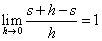h is a 3d number

A consequence is: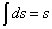The derivative of s2: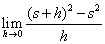h is a 3d number

But, because the algebra is not distributive (s + h)2 ≠ s2 + h2 + 2. s.h , simulations shows the limit does not exist (see code). So the integral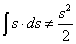depends on the path.

Anyway, if we have a point in the space

6)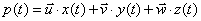(t ∈ℜ)

Where x(t), y(t), z(t) gives a real result and are continuous and differentiable functions, we can calculate the velocity: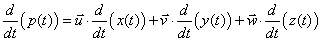and also the acceleration.

If we have a sum of two 3d functions such as 6):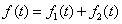(t ∈ℜ)

The derivative is:

7)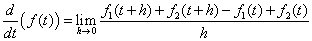And can be expressed as the sum of the two derivatives; limit 7) is: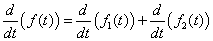(t ∈ℜ)

Instead, if we have a product of two 3d functions such as 6):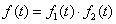(t ∈ℜ)

The derivative is:

8)(t,h∈ℜ) This algebra is not distributive, so the limit can’t be expressed in the algebraic well known form: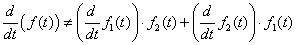But, if the limit exists, can be calculated.

I suppose that if f1(t) and f2(t) are continuous and differentiable functionsI, the limit 8) exists and can be calculated, but it is not an easy demonstration.

lThe limitation is (x(t), y(t), z(t)) must be a real term.

t can be a complex 2d variable, but the functions must be continuous and differentiable and their result must be a real number.

#### Last Considerations about 4d Numbers (see)

If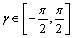during calculations, same considerations can be done for 4d numbers (except for sin(s) and cos(s) that in this case they don’t to be take in consideration).

If during calculations happens that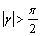we have a problem, the transformations between cartesian notation and vector notation, in this case, are not perfectly biunique.

When cos(γ)<0, the 4d number, become a number that has a negative 3d space sign: r' = r.cos(γ)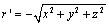and the sign is negative because cos(γ)<0

We can take into account of the sign of the 3d space so we can have a perfectly biunique transformation.

For the product definition there is no problem, but we have problems with the sum definition. May be a nonsense make a sum between two 4d numbers, one with a sign of 3d space > 0 and one with a negative sign of the 3d space.

I make a proposal:

If the 3d space signs of a and b are the same, the sum is the standard sum.

Suppose, instead that 3d space sign of a and b are different.

Let us consider a and b two 4d numbers whose t value is 0, same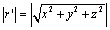but opposite 3d spaces.

We can say the sum (a+b) of this two numbers reduces to 0, just like a difference. So, in this special case, the sum reduces to a difference, and the resulting sign of the 3d space is set to 1.

In general we can have r'a≠r'b. We can built up a function that take into account that r' can be negative, then make a+b among the new deduced (x,y,z,t) components; the resulting sum sign of the 3d space is the a 3d space sign if r'a> r'b; if r'a< r'b the resulting sum sign of the 3d space is the b 3d space sign.

Because: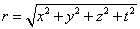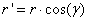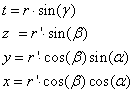The function that take into account that r' can be negative is very simple: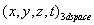>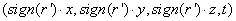(See appendix for the code Sum_4d for the sum definition).

Test code:

This is the test code that permit to simulate the calculus of(h is a 3d number)

Function Test1(s As Complex3d, h As Complex3d) As Complex3d

Dim a As Complex3d, b As Complex3d, b_minus_a As Complex3d

b = Sum_3d(s, h) ‘s+h

a = Mul_3d(s, s) ' s^2

b = Mul_3d(b, b) '(s+h)^2

b_minus_a = Diff_3d(b, a) '(s+h)^2 - s^2

Test1 = Div_3d(b_minus_a, h)

End Function

This is the test code that permit to simulate the calculus of(h is a 3d number)

Function Test 2 (s As Complex3d, h As Complex3d) As Complex3d

Dim a As Complex3d, b As Complex3d, b_minus_a As Complex3d

b = Sum_3d(s, h) ' s+h

a = Exp_3d(s) ' Exp(s)

b = Exp_3d(b) ' Exp(s+h)

b_minus_a = Diff_3d(b, a) 'Exp(s+h) - Exp(s)

Test2 = Div_3d(b_minus_a, h)

End Function

'-----------------------------------

es and ln(s) code functions:

'-----------------------------------

Function Exp_3d(S As Complex3d) As Complex3d

Dim Es As Complex3d

Es = Init_Vector(Exp(S.X), Modulus(S.Y, 2 * Pi), Modulus(S.Z, 2 * Pi))

Exp_3d = Es

End Function

Function Log_3d(S As Complex3d) As Complex3d

Log_3d = Init_Algebric(Log(S.R), S.Alfa, S.Beta)

End Function

'-----------------------------------

3D core algebra: visual basic source code

Option Compare Database

Option Explicit

'-----------------------------------

' CORE 3d ALGEBRA

' V2.4g OPTIMIZED

'-----------------------------------

Public Const Pi = 3.14159265358979

'------------------------------------------------------------------------

'AVOID THE USE OF SMALL NUMBER IN SIMULATION (OR VERY BIG NUMBERS) THE PRECISION IS LIMITED, THE MANTISSA HAVE 15 DIGIT

Public Const MaxDigit = 12, AsZero = 10 ^ -12

'We can round the results of calculus or not

Private Const Round_Results = True

'-----------------------------------------------------------------------

'The definition of the complex3d type

Type Complex3d

X As Double

Y As Double

Z As Double

R As Double

Alfa As Double

Beta As Double

End Type

'The initialization number in cartesian notation

Function Init_Algebric(X As Double, Y As Double, Z As Double) As Complex3d

Dim R As Complex3d

R.X = X

R.Y = Y

R.Z = Z

Calc_Vector_Notation R

Init_Algebric = R

End Function

'The initialization number in vector notation

Function Init_Vector(R As Double, Alfa As Double, Beta As Double) As Complex3d

Dim S As Complex3d

S.R = R

S.Alfa = Alfa

S.Beta = Beta

To_Algebric_Notation S

Init_Vector = S

End Function

'The sum A+B

Function Sum_3d(a As Complex3d, b As Complex3d) As Complex3d

Dim R As Complex3d

R.X = a.X + b.X

R.Y = a.Y + b.Y

R.Z = a.Z + b.Z

Calc_Vector_Notation R

Sum_3d = R

End Function

'The difference A-B

Function Diff_3d(a As Complex3d, b As Complex3d) As Complex3d

Dim R As Complex3d

R.X = a.X - b.X

R.Y = a.Y - b.Y

R.Z = a.Z - b.Z

Calc_Vector_Notation R

Diff_3d = R

End Function

'The product A*B

Function Mul_3d(a As Complex3d, b As Complex3d) As Complex3d

Dim R As Complex3d

R.R = a.R * b.R

R.Alfa = Modulus(a.Alfa + b.Alfa, 2 * Pi)

R.Beta = Modulus(a.Beta + b.Beta, 2 * Pi)

To_Algebric_Notation R

Mul_3d = R

End Function

'The division A/B

Function Div_3d(a As Complex3d, b As Complex3d) As Complex3d

Dim R As Complex3d

R.R = a.R / b.R

R.Alfa = Modulus(a.Alfa - b.Alfa, 2 * Pi)

R.Beta = Modulus(a.Beta - b.Beta, 2 * Pi)

To_Algebric_Notation R

Div_3d = R

End Function

'The 1/S

Function Inverse_3d(S As Complex3d) As Complex3d

Dim R As Complex3d

R.R = 1 / S.R

R.Alfa = Modulus(-S.Alfa, 2 * Pi)

R.Beta = Modulus(-S.Beta, 2 * Pi)

To_Algebric_Notation R

Inverse_3d = R

End Function

'S^X; X real

Function S_elev_X_3d(S As Complex3d, X As Double) As Complex3d

Dim R As Complex3d

R.R = S.R ^ X

R.Alfa = Modulus(S.Alfa * X, 2 * Pi)

R.Beta = Modulus(S.Beta * X, 2 * Pi)

To_Algebric_Notation R

S_elev_X_3d = R

End Function

'Square root of S

Function Sqr_3d(S As Complex3d) As Complex3d

Dim R As Complex3d

R.R = Sqr(S.R)

R.Alfa = Modulus(S.Alfa / 2, 2 * Pi)

R.Beta = Modulus(S.Beta / 2, 2 * Pi)

To_Algebric_Notation R

Sqr_3d = R

End Function

'Rotation and elongation

Function Rotation_3d(S As Complex3d, dAlfa As Double, dBeta As Double, Optional dr As Double = 0) As Complex3d

Dim R As Complex3d

R = S

If Near0(R.R) = 0 And Near0(dr) = 0 Then

Rotation_3d = R

Exit Function

End If

R.R = R.R + dr

R.Alfa = Modulus(S.Alfa + dAlfa, 2 * Pi)

R.Beta = Modulus(S.Beta + dBeta, 2 * Pi)

To_Algebric_Notation R

Rotation_3d = R

End Function

'Creates ds from a vector S and dAlfa, dBeta and dr

Function Differentiate_Vector_3d(S As Complex3d, dAlfa As Double, dBeta As Double, dr As Double) As Complex3d

Dim dx As Double, dy As Double, dz As Double, dS As Complex3d

dz = dr * Sin(S.Beta) + S.R * Cos(S.Beta) * dBeta

dy = dr * Cos(S.Beta) * Sin(S.Alfa) - S.R * Sin(S.Beta) * Sin(S.Alfa) * dBeta + S.R * Cos(S.Beta) * Cos(S.Alfa) * dAlfa

dx = dr * Cos(S.Beta) * Cos(S.Alfa) - S.R * Sin(S.Beta) * Cos(S.Alfa) * dBeta - S.R * Cos(S.Beta) * Sin(S.Alfa) * dAlfa

dS = Init_Algebric(dx, dy, dz)

Differentiate_Vector_3d = dS

End Function

'Internal product

Function A_V_B_3d(a As Complex3d, b As Complex3d) As Double

A_V_B_3d = a.X * b.X + a.Y * b.Y + a.Z * b.Z

End Function

'External product

Function A_X_B_3d(a As Complex3d, b As Complex3d) As Complex3d

Dim X As Double, Y As Double, Z As Double, R As Complex3d

X = a.Y * b.Z - a.Z * b.Y

Y = a.Z * b.X - a.X * b.Z

Z = a.X * b.Y - a.Y * b.X

R = Init_Algebric(X, Y, Z)

A_X_B_3d = R

End Function

'Versor of S

Function Versor_3d(S As Complex3d) As Complex3d

Dim R As Complex3d, R0 As Double

If Near0(S.R) = 0 Then GoTo Set_To_Zero

R = S

R0 = R.R

R.R = 1

R.X = R.X / R0

R.Y = R.Y / R0

R.Z = R.Z / R0

Check_Algebric_Zero R ‘may be omitted

Versor_3d = R

Exit Function

Set_To_Zero:

R.X = 0

R.Y = 0

R.Z = 0

R.R = 0

R.Alfa = 0

R.Beta = 0

Versor_3d = R

End Function

'Return vector A along components on B axes; B new real axes

Function Project_A_on_B_3d(a As Complex3d, b As Complex3d) As Complex3d

Dim Wx As Complex3d, Wy As Complex3d, Wz As Complex3d, R As Complex3d, R0 As Double

Dim X As Double, Y As Double, Z As Double

Dim BVx As Double, BVy As Double, BVz As Double

If Near0(b.R) = 0 Then GoTo Set_To_Zero

If Near0(a.R) = 0 Then GoTo Set_To_Zero

'Versors Wx, Wy and Wz the new base

Wx = Versor_3d(b)

'----------------------------------------------------

' Optimization

'Wy = Init_Algebric_3d(-Wy.Y, Wy.X, 0)

Wy.X = -Wx.Y

Wy.Y = Wx.X

Wy.Z = 0

R0 = Sqr(Wy.X ^ 2 + Wy.Y ^ 2)

If Near0(R0) = 0 Then R0 = 1 ‘ this do not stop the calculation

Wy.X = Wy.X / R0

Wy.Y = Wy.Y / R0

'----------------------------------------------------

''----------------------------------------------------

'consider Wz as

'Wz = A_X_B_3d(Wx, Wy)

Wz.X = Wx.Y * Wy.Z - Wx.Z * Wy.Y

Wz.Y = Wx.Z * Wy.X - Wx.X * Wy.Z

Wz.Z = Wx.X * Wy.Y - Wx.Y * Wy.X

'----------------------------------------------------

''Project A on Wx, Wy, Wz, Wt

BVx = A_V_B_3d(a, Wx)

BVy = A_V_B_3d(a, Wy)

BVz = A_V_B_3d(a, Wz)

R = Init_Algebric(BVx, BVy, BVz)

Project_A_on_B_3d = R

Exit Function

Set_To_Zero:

R.X = 0

R.Y = 0

R.Z = 0

R.R = 0

R.Alfa = 0

R.Beta = 0

Project_A_on_B_3d = R

End Function

'The Transformation from Cartesian to Vector Notation

Private Sub Calc_Vector_Notation(S As Complex3d)

Dim SinBeta As Double, CosBeta As Double, SinAlfa As Double, CosAlfa As Double

Check_Algebric_Zero S

'Calc R

S.R = Sqr(S.X ^ 2 + S.Y ^ 2 + S.Z ^ 2)

If Near 0(S.R) = 0 Then GoTo Set_To_Zero

'Solve Beta....

SinBeta = S.Z / S.R

CosBeta = Sqr(S.X ^ 2 + S.Y ^ 2) / S.R

'CosBeta is always >=0

'SinBeta can be <=0

If Round(CosBeta, MaxDigit) = 0 Then

If Round (SinBeta, MaxDigit) = 0 Then GoTo Set_To_Zero

S.Beta = Pi / 2 * Sgn(S.Z)

S.Alfa = 0

Exit Sub

End If

S.Beta = ArcSin(SinBeta)

'Solve Alfa....

SinAlfa = S.Y / S.R / CosBeta

CosAlfa = S.X / S.R / CosBeta

'CosAlfa can be <=0 ...

If Round (CosAlfa, MaxDigit) = 0 Then

If Round (SinAlfa, MaxDigit) = 0 Then

S.Alfa = 0

Else

S.Alfa = Pi / 2 * Sgn(S.Y)

End If

Else

S.Alfa = ArcSin(SinAlfa)

If CosAlfa < 0 Then

'If CosAlfa<0 ... -> Quadrant 2 o quadrant 4

If Near 0 (S.Alfa) <> 0 Then

S.Alfa = (Pi – Abs (S.Alfa)) * Sgn(S.Y)

Else

S.Alfa = Pi

End If

End If

End If

Exit Sub

Set_To_Zero:

S.X = 0

S.Y = 0

S.Z = 0

S.R = 0

S.Alfa = 0

S.Beta = 0

End Sub

'The Transformation from Vector to Cartesian Notation

Private Sub To_Algebric_Notation(S as Complex3d)

Dim CosBeta as Double

If Near0(S.R) = 0 Then GoTo Set_To_Zero

'Solve X,Y,Z

S.Z = S.R * Sin(S.Beta)

CosBeta = Cos(S.Beta)

If Near0(CosBeta) = 0 Then

S.Y = 0

S.X = 0

'If CosBeta=0 Alfa is irrelevant

S.Alfa = 0

Else

S.Y = S.R * CosBeta * Sin(S.Alfa)

S.X = S.R * CosBeta * Cos(S.Alfa)

End If

Calc_Vector_Notation S

Exit Sub

Set_To_Zero:

S.X = 0

S.Y = 0

S.Z = 0

S.R = 0

S.Alfa = 0

S.Beta = 0

End Sub

Private Sub Check_Algebric_Zero(S as Complex3d)

S.Z = Near0(S.Z)

S.Y = Near0(S.Y)

S.X = Near0(S.X)

End Sub

Function Modulus(X as Double, Y As Double) As Double

Dim Resto As Double

Resto = X / Y - Fix(X / Y)

If Round(Resto * Y, MaxDigit) = 0 Then

Modulus = 0

Else

Modulus = Resto * Y

End If

End Function

Function ArcSin(X as Double) As Double

If Round(Abs(X), MaxDigit) = 1 Then

ArcSin = Pi / 2 * Sgn(X)

Exit Function

End If

ArcSin = Atn(X / Sqr(1 - X ^ 2))

End Function

Function ArcCos(X as Double) As Double

If Round(Abs(X), MaxDigit) = 1 Then

If X > 0 Then

ArcCos = 0

Exit Function

Else

ArcCos = Pi

Exit Function

End If

End If

ArcCos = Atn(-X / Sqr(1 - X ^ 2)) + 2 * Atn(1)

End Function

Function Near0(X As Double) As Double

Dim R As Double

R = X

If Round_Results Then R = Round(R, MaxDigit)

Near0 = R

If Abs(R) <= AsZero Then

Near0 = 0

End If

End Function

Function Truncate(X as Double, MaxDigit) As Double

Dim R as Double

R = X * 10 ^ MaxDigit

'The mantissa...

R = Fix(R)

R = R / 10 ^ MaxDigit

Truncate = R

End Function

'-----------------------------------

'END CORE 3d ALGEBRA

'-----------------------------------

4D core algebra: visual basic source code

Option Compare Database

Option Explicit

'-----------------------------------

' CORE 4d ALGEBRA

' V2.8e OPTIMIZED

'-----------------------------------

'Public Const Pi = 3.14159265358979

'----------------------------------------------

'AVOID THE USE OF SMALL NUMBER IN SIMULATION (OR VERY BIG NUMBERS) THE PRECISION IS LIMITED, THE MANTISSA HAVE 15 DIGIT

'Public Const MaxDigit = 12, AsZero = 10 ^ -12

'We can round the results of calculus or not

Private Const Round_Results = True

'----------------------------------------------

'The definition of the complex4d type

Type Complex4d

X As Double

Y As Double

Z As Double

T As Double

R As Double

Alfa As Double

Beta As Double

Gamma As Double

V3dSpace As Integer

End Type

'The initialization number in cartesian notation

Function Init_Algebric_4d(X As Double, Y As Double, Z As Double, T As Double, V3dSpace As Integer) As Complex4d

Dim R As Complex4d

R.X = X

R.Y = Y

R.Z = Z

R.T = T

R.V3dSpace = V3dSpace

Calc_Vector_Notation R

Init_Algebric_4d = R

End Function

'The initialization number in vector notation

Function Init_Vector_4d(R As Double, Alfa As Double, Beta As Double, Gamma As Double) As Complex4d

Dim S As Complex4d

S.R = R

S.Alfa = Alfa

S.Beta = Beta

S.Gamma = Gamma

To_Algebric_Notation S

Init_Vector_4d = S

End Function

'The sum A+B

Function Sum_4d(a As Complex4d, b As Complex4d) As Complex4d

Dim r1a As Double, r1b As Double, R As Complex4d

'Same 3d spaces

If a.V3dSpace = b.V3dSpace Then

'Standard sum

R.X = a.X + b.X

R.Y = a.Y + b.Y

R.Z = a.Z + b.Z

R.T = a.T + b.T

R.V3dSpace = a.V3dSpace

Calc_Vector_Notation R

Sum_4d = R

Exit Function

End If

'Consider r1a and r1b

r1a = Sqr(a.X ^ 2 + a.Y ^ 2 + a.Z ^ 2)

r1b = Sqr(b.X ^ 2 + b.Y ^ 2 + b.Z ^ 2)

'Make the sum:

R.X = a.V3dSpace * a.X + b.V3dSpace * b.X

R.Y = a.V3dSpace * a.Y + b.V3dSpace * b.Y

R.Z = a.V3dSpace * a.Z + b.V3dSpace * b.Z

R.T = a.T + b.T

If r1a = r1b Then 'this is a limit case. R.V3d space is set to 1 by default

R.V3dSpace = 1 'this choice may be questionable

Calc_Vector_Notation R

Sum_4d = R

Exit Function

End If

If r1a > r1b Then

R.V3dSpace = a.V3dSpace

Else

R.V3dSpace = b.V3dSpace

End If

Calc_Vector_Notation R

Sum_4d = R

End Function

'The difference A-B

Function Diff_4d(a As Complex4d, b As Complex4d) As Complex4d

Dim b1 As Complex4d

'consider the simmetric vector:

b1 = Init_Algebric_4d(-b.X, -b.Y, -b.Z, -b.T, b.V3dSpace)

Diff_4d = Sum_4d(a, b1)

End Function

'The product A*B

Function Mul_4d(a As Complex4d, b As Complex4d) As Complex4d

Dim R As Complex4d

R.R = a.R * b.R

R.Alfa = Modulus(a.Alfa + b.Alfa, 2 * Pi)

R.Beta = Modulus(a.Beta + b.Beta, 2 * Pi)

R.Gamma = Modulus(a.Gamma + b.Gamma, 2 * Pi)

To_Algebric_Notation R

Mul_4d = R

End Function

'The division A/B

Function Div_4d(a As Complex4d, b As Complex4d) As Complex4d

Dim R As Complex4d

R.R = a.R / b.R

R.Alfa = Modulus(a.Alfa - b.Alfa, 2 * Pi)

R.Beta = Modulus(a.Beta - b.Beta, 2 * Pi)

R.Gamma = Modulus(a.Gamma - b.Gamma, 2 * Pi)

To_Algebric_Notation R

Div_4d = R

End Function

'The 1/S

Function Inverse_4d(S As Complex4d) As Complex4d

Dim R As Complex4d, X As Double

R.R = 1 / S.R

R.Alfa = Modulus(-S.Alfa, 2 * Pi)

R.Beta = Modulus(-S.Beta, 2 * Pi)

R.Gamma = Modulus(-S.Gamma, 2 * Pi)

To_Algebric_Notation R

Inverse_4d = R

End Function

'S^X; X real

Function S_elev_X_4d(S As Complex4d, X As Double) As Complex4d

Dim R As Complex4d

R.R = S.R ^ X

R.Alfa = Modulus(S.Alfa * X, 2 * Pi)

R.Beta = Modulus(S.Beta * X, 2 * Pi)

R.Gamma = Modulus(S.Gamma * X, 2 * Pi)

To_Algebric_Notation R

S_elev_X_4d = R

End Function

'Square root of S

Function Sqr_4d(S As Complex4d) As Complex4d

Dim R As Complex4d

R.R = Sqr(S.R)

R.Alfa = Modulus(S.Alfa / 2, 2 * Pi)

R.Beta = Modulus(S.Beta / 2, 2 * Pi)

R.Gamma = Modulus(S.Gamma / 2, 2 * Pi)

To_Algebric_Notation R

Sqr_4d = R

End Function

'Rotation and elongation

Function Rotation_4d(S As Complex4d, dAlfa As Double, dBeta As Double, dGamma As Double, Optional dr As Double = 0) As Complex4d

Dim R As Complex4d

R = S

If Near0(R.R) = 0 And Near0(dr) = 0 Then

Rotation_4d = R

Exit Function

End If

R.R = R.R + dr

R.Alfa = Modulus(S.Alfa + dAlfa, 2 * Pi)

R.Beta = Modulus(S.Beta + dBeta, 2 * Pi)

R.Gamma = Modulus(S.Gamma + dGamma, 2 * Pi)

To_Algebric_Notation R

Rotation_4d = R

Exit Function

End If

R.R = R.R + dr

R.Alfa = Modulus(S.Alfa + dAlfa, 2 * Pi)

R.Beta = Modulus(S.Beta + dBeta, 2 * Pi)

R.Gamma = Modulus(S.Gamma + dGamma, 2 * Pi)

To_Algebric_Notation R

Rotation_4d = R

End Function

'Creates ds from a vector S and dAlfa, dBeta, dGamma and dr

Function Differentiate_Vector_4d(S As Complex4d, dAlfa As Double, dBeta As Double, dGamma As Double, dr As Double) As Complex4d

Dim dx As Double, dy As Double, dz As Double, dt As Double, ds As Complex4d

Dim dr1 As Double, R1 As Double

R1 = Sqr(S.X ^ 2 + S.Y ^ 2 + S.Z ^ 2)

dr1 = dr * Cos(S.Gamma) - S.R * Sin(S.Gamma) * dGamma

dt = dr * Sin(S.Gamma) + S.R * Cos(S.Gamma) * dGamma

dz = dr1 * Sin(S.Beta) + S.R * Cos(S.Beta) * dBeta

dy = dr1 * Cos(S.Beta) * Sin(S.Alfa) - S.R * Sin(S.Beta) * Sin(S.Alfa) * dBeta + S.R * Cos(S.Beta) * Cos(S.Alfa) * dAlfa

dx = dr1 * Cos(S.Beta) * Cos(S.Alfa) - S.R * Sin(S.Beta) * Cos(S. Alfa) * dBeta - S.R * Cos(S.Beta) * Sin(S.Alfa) * dAlfa

ds = Init_Algebric_4d(dx, dy, dz, dt, S.V3dSpace)

Differentiate_Vector_4d = ds

End Function

'Proposal function - nonsense?

'Internal product

Function A_V_B_4d(a As Complex4d, b As Complex4d) As Double

A_V_B_4d = a.V3dSpace * a.X * b.V3dSpace * b.X + _

a.V3dSpace * a.Y * b.V3dSpace * b.Y + _

a.V3dSpace * a.Z * b.V3dSpace * b.Z + _

a.T * b.T

End Function

'Versor of S

Function Versor_4d(S As Complex4d) As Complex4d

Dim R As Complex4d, R0 As Double

If Near0(S.R) = 0 Then GoTo Set_To_Zero

R = S

R0 = R.R

R.R = 1

R.X = R.X / R0

R.Y = R.Y / R0

R.Z = R.Z / R0

R.T = R.T / R0

R.V3dSpace = S.V3dSpace

Check_Algebric_Zero_4d R

Versor_4d = R

Exit Function

Set_To_Zero:

R.X = 0

R.Y = 0

R.Z = 0

R.T = 0

R.R = 0

R.Alfa = 0

R.Beta = 0

R.Gamma = 0

R.V3dSpace = 1

Versor_4d = R

End Function

'Proposal function -- may be a nonsense

'Return vector A along components on B axes; B new real axes

Function Project_A_on_B_4d(a As Complex4d, b As Complex4d) As Complex4d

Dim Wx As Complex4d, Wy As Complex4d, Wz As Complex4d, Wt As Complex4d, R As Complex4d, R0 As Double

Dim X As Double, Y As Double, Z As Double, T As Double

Dim BVx As Double, BVy As Double, BVz As Double, BVt As Double

If Near0(b.R) = 0 Then GoTo Set_To_Zero

If Near0(a.R) = 0 Then GoTo Set_To_Zero

'Versors Wx, Wy and Wz the new base

Wx = Versor_4d(b)

'----------------------------------------------------

' Optimization

'Wy = Init_Algebric_4d(-Wy.Y, Wy.X, 0,0)

Wy.X = -Wx.Y

Wy.Y = Wx.X

Wy.Z = 0

Wy.T = 0

Wy.V3dSpace = b.V3dSpace

'Wy = Versor_4d(Wy)

R0 = Sqr(Wy.X ^ 2 + Wy.Y ^ 2 + Wy.Z ^ 2 + Wy.T ^ 2)

If Near0(R0) = 0 Then R0 = 1 'this do not stop the calculation

Wy.X = Wy.X / R0

Wy.Y = Wy.Y / R0

'Wy.Z = Wy.Z / R0

'Wy.T = Wy.T / R0

'----------------------------------------------------

''----------------------------------------------------

'consider Wz as

'Wz = A_X_B_3d(Wx, Wy)+ T=0

Wz.X = Wx.Y * Wy.Z - Wx.Z * Wy.Y

Wz.Y = Wx.Z * Wy.X - Wx.X * Wy.Z

Wz.Z = Wx.X * Wy.Y - Wx.Y * Wy.X

Wz.T = 0

Wz.V3dSpace = b.V3dSpace

'----------------------------------------------------

''Wt: Take Wx and make it ortogonal respect to T

If Near0(Wx.T) = 0 Then

Wt.X = 0

Wt.Y = 0

Wt.Z = 0

Wt.T = 0

Wt.V3dSpace = 1

Else

Wt.X = Wx.X

Wt.Y = Wx.Y

Wt.Z = Wx.Z

Wt.T = -(Wx.X ^ 2 + Wx.Y ^ 2 + Wx.Z ^ 2) / Wx.T

Wt.V3dSpace = b.V3dSpace

End If

'Wt = Versor_4d(Wt)

R0 = Sqr(Wt.X ^ 2 + Wt.Y ^ 2 + Wt.Z ^ 2 + Wt.T ^ 2)

If Near0(R0) = 0 Then R0 = 1 'this do not stop the calculation

Wt.X = Wt.X / R0

Wt.Y = Wt.Y / R0

Wt.Z = Wt.Z / R0

Wt.T = Wt.T / R0

'Project A on Wx, Wy, Wz, Wt

BVx = A_V_B_4d(a, Wx)

BVy = A_V_B_4d(a, Wy)

BVz = A_V_B_4d(a, Wz)

BVt = A_V_B_4d(a, Wt)

R = Init_Algebric_4d(BVx, BVy, BVz, BVt, b.V3dSpace) ' or (?)

'R = Init_Algebric_4d(BVx, BVy, BVz, BVt, a.V3dSpace)

Project_A_on_B_4d = R

Exit Function

Set_To_Zero:

R.X = 0

R.Y = 0

R.Z = 0

R.T = 0

R.R = 0

R.Alfa = 0

R.Beta = 0

R.Gamma = 0

R.V3dSpace = 1

Project_A_on_B_4d = R

End Function

'The Transformation from Cartesian to Vector Notation

Private Sub Calc_Vector_Notation(S As Complex4d)

Dim S3 As Complex3d, SinGamma As Double, CosGamma As Double, R1 As Double

Dim SinBeta As Double, CosBeta As Double, SinAlfa As Double, CosAlfa As Double

Check_Algebric_Zero_4d S

'Calc R

S.R = Sqr(S.X ^ 2 + S.Y ^ 2 + S.Z ^ 2 + S.T ^ 2)

If Near0(S.R) = 0 Then GoTo Set_To_Zero

R1 = Sqr(S.X ^ 2 + S.Y ^ 2 + S.Z ^ 2)

'Solve Gamma....

SinGamma = S.T / S.R

'SinGamma can be <=0

CosGamma = S.V3dSpace * R1 / S.R

'CosGamma can be<0 depends on V3dSpace

If Round(CosGamma, MaxDigit) = 0 Then '->R1=0; considerate T

If Round(SinGamma, MaxDigit) = 0 Then GoTo Set_To_Zero 'i.e. T=0, and R1=0

S.Gamma = Pi / 2 * Sgn(S.T)

Else

S.Gamma = ArcSin(SinGamma)

If S.V3dSpace < 0 Then

If Near0(S.T) <> 0 Then

S.Gamma = (Pi - Abs(S.Gamma)) * Sgn(S.T)

Else

S.Gamma = Pi

End If

End If

End If

If Near0(R1) = 0 Then 'pure T vector

S.X = 0

S.Y = 0

S.Z = 0

S.Alfa = 0

S.Beta = 0

S.Gamma = Pi / 2 * Sgn(S.T)

S.V3dSpace = 1

Exit Sub

End If

'Solve X,Y,Z

'Solve Beta....

SinBeta = S.Z / R1

CosBeta = Sqr(S.X ^ 2 + S.Y ^ 2) / R1

'CosBeta is always >=0

'SinBeta can be <=0

If Round(CosBeta, MaxDigit) = 0 Then If Round(SinBeta, MaxDigit) = 0 Then GoTo Set_To_Zero

S.Beta = Pi / 2 * Sgn(S.Z)

S.Alfa = 0

Exit Sub

End If

S.Beta = ArcSin(SinBeta)

'Solve Alfa....

SinAlfa = S.Y / R1 / CosBeta

CosAlfa = S.X / R1 / CosBeta

'CosAlfa can be <=0 ...

If Round(CosAlfa, MaxDigit) = 0 Then

If Round(SinAlfa, MaxDigit) = 0 Then

S.Alfa = 0

Else

S.Alfa = Pi / 2 * Sgn(S.Y)

End If

Else

S.Alfa = ArcSin(SinAlfa)

If CosAlfa < 0 Then

'If CosAlfa<0 ... -> Quadrant 2 o quadrant 4

If Near0(S.Alfa) <> 0 Then

S.Alfa = (Pi - Abs(S.Alfa)) * Sgn(S.Y)

Else

S.Alfa = Pi

End If

End If

End If

Exit Sub

Set_To_Zero:

S.X = 0

S.Y = 0

S.Z = 0

S.R = 0

S.Alfa = 0

S.Beta = 0

S.Gamma = 0

S.V3dSpace = 1

End Sub

'The Transformation from Vector to Cartesian Notation

Private Sub To_Algebric_Notation(S As Complex4d)

Dim R1 As Double, CosBeta As Double, CosGamma As Double

If Near0(S.R) = 0 Then GoTo Set_To_Zero

'Solve X,Y,Z, T

S.T = S.R * Sin(S.Gamma)

CosGamma = Cos(S.Gamma)

If Near0(CosGamma) = 0 Then

'The Vector is a pure T vector, so

S.Z = 0

S.Y = 0

S.X = 0

'Alfa and Beta irrelevant, set to 0

S.Beta = 0

S.Alfa = 0

S.Gamma = Pi / 2 * Sgn(S.T)

S.V3dSpace = 1

Exit Sub

End If

S.V3dSpace = Sgn(CosGamma)

R1 = S.R * Abs(CosGamma)

S.Z = R1 * Sin(S.Beta)

CosBeta = Cos(S.Beta)

If Near0(CosBeta) = 0 Then

S.Y = 0

S.X = 0

'If CosBeta=0 Alfa is irrelevant

S.Alfa = 0

Else

S.Y = R1 * CosBeta * Sin(S.Alfa)

S.X = R1 * CosBeta * Cos(S.Alfa)

End If

Calc Vector Notation S

Exit Sub

Set_To_Zero:

S.X = 0

S.Y = 0

S.Z = 0

S.T = 0

S.R = 0

S.Alfa = 0

S.Beta = 0

S.Gamma = 0

S.V3dSpace = 1

End Sub

Private Sub Check_Algebric_Zero_4d(S As Complex4d)

S.Z = Near0(S.Z)

S.Y = Near0(S.Y)

S.X = Near0(S.X)

S.T = Near0(S.T)

If Near0(Sqr(S.X ^ 2 + S.Y ^ 2 + S.Z ^ 2 + S.T ^ 2)) = 0 Then S.V3dSpace = 1

End Sub

'-----------------------------------

'END CORE 4d ALGEBRA

'-----------------------------------

#### References

Select your language of interest to view the total content in your interested language

### Article Usage

• Total views: 8310
• [From(publication date):
December-2015 - Aug 21, 2019]
• Breakdown by view type
• HTML page views : 8213
• PDF downloads : 97

## Post your commentCan't read the image? click here to refresh
###### Peer Reviewed Journals

Make the best use of Scientific Research and information from our 700 + peer reviewed, Open Access Journals

International Conferences 2019-20

Meet Inspiring Speakers and Experts at our 3000+ Global Annual Meetings

Top